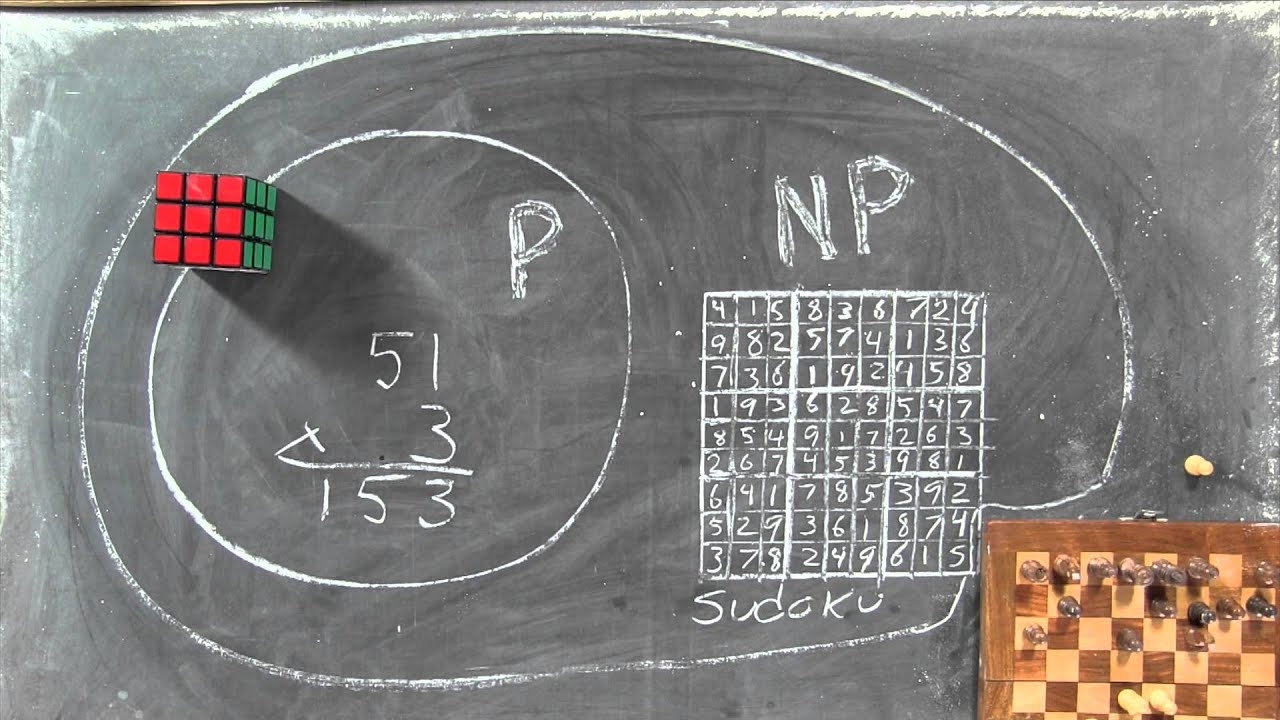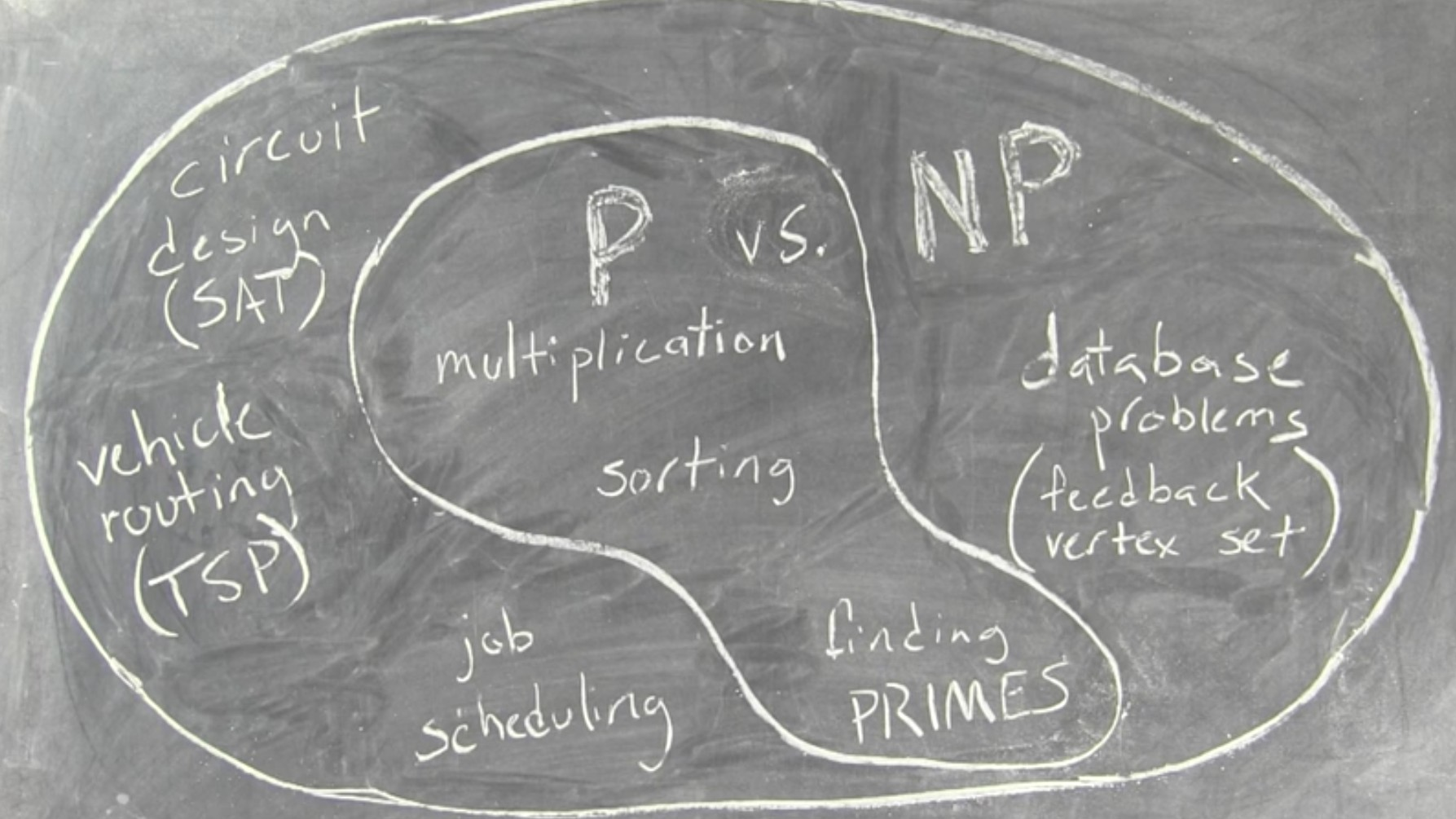×

Search anything:

# Approximate algorithms for NP problems

#### Algorithms Time Complexity Approximation AlgorithmGet this book -> Problems on Array: For Interviews and Competitive Programming

In this article, we have covered Idea of Approximate algorithms for NP problems. NP problems are tough but Approximate algorithms are considered to be a good approach as we get a answer close to the real answer in reasonable time. We have covered the basics with examples of problems like Bin Packing.

Table of content:

1. Introduction to P, NP, NP-Hard and more
2. Approximation algorithms for NP
3. The quality of an approximation
4. Approximation algorithms with small additive error
5. Problems having polynomial approximation schemes
6. Optimization problems with constant-factor approximations
7. Hard-to-approximate problems

# Introduction to P, NP, NP-Hard and more

Take a look at this picture which captures the complete idea:P:

• The class of problems that have polynomial-time deterministic algorithms. (solvable in a reasonable amount of time)
• Set of problems that can be solved in polynomial time.

NP:

• The class of problems that are solvable in polynomial time on a non-deterministic algorithms.
• Set of problems for which a solution can be verified in polynomial time.

P VS NP

• If the solution to a problem is easy to check for correctness, must the problem be easy to solve?
• P is subset of NP. Any problem that can be solved by deterministic machine in polynomial time can also be solved by non-deterministic machine in polynomial time.

NP - HARD

• Problems that are "at least as hard as the hardest problems in NP".

NP-completeness

• Problems for which the correctness of each solution can be verified quickly and a brute-force search algorithm can actually find a solution by trying all possible solutions.# Approximation algorithms for NP

• Approximation algorithms are efficient algorithms that find approximate solutions to optimization problems with provable guarantees on the distance of the returned solution to the optimal one.

• An optimization problem consists in finding the best (cheapest, heaviest, etc.) element in a large set P, called the feasible region.

• Many problems of practical significance are NP-complete but are too important to abandon merely because obtaining an optimal solution is intractable.

• If a problem is NP-complete, we are unlikely to find a polynomial-time algorithm for solving it exactly, but this does not imply that all hope is lost.

• There are two approaches to getting around NP-completeness. First, if the actual inputs are small, an algorithm with exponential running time may be perfectly satisfactory.

• Second, it may still be possible to find near-optimal solutions in polynomial time (either in the worst case or on the average). In practice, near-optimality is often good enough. An algorithm that returns near-optimal solutions is called an approximation algorithm.

• Depending on the problem, an optimal solution may be defined as one with maximum possible cost or one with minimum possible cost; the problem may be a maximization or a minimization problem.

# The quality of an approximation

• In any combinatorial optimization problem, there is some objective function we are supposed to optimize.

• The approximation ratio (or approximation factor) of an algorithm is the ratio between the result obtained by the algorithm and the optimal cost or profit.

• Typically this ratio is taken in whichever direction makes it bigger than one.

• An algorithm with approximation ratio k is called a k-approximation algorithm.

• When the approximation ratio is close to 1, it is often more useful to look at the approximation error, which is defined as the approximation ratio minus 1.

• A family of algorithms that can achieve any constant approximation error in polynomial time is called a polynomial-time approximation scheme or PTAS.

# Approximation algorithms with small additive error

To illustrate Approximation algorithms with small additive error, we have covered 3 problems:

1. Edge Coloring
2. Bin Packing
3. Randomized rounding and linear programming

### Edge Coloring

• Edge Coloring: Given a graph, color its edges with a minimum number of colors so that, for each vertex, the edges incident to that vertex are all different colors.

• For this problem, it is easy to find a witness.

• For any graph G, let v be the vertex of highest degree in G.

• Clearly one needs to assign at least degG(v) colors to the edges of G, for otherwise there would be two edges with the same color incident to v.

• For any graph G, there is an edge coloring using a number of colors equal
to one plus the degree of G.

• The proof of this fact translates into a polynomial-time algorithm that approximates the minimum edge-coloring to within an additive error of 1.

• Most efficient algorithm which edge-colors a simple graph G with n vertices and m edges, and at most `A(G) + 1` colors in `O(m * sqrt(nlogn))` time.

• Here A(G) represents maximum degree of graph G.

• For a multigraph time complexity would be `O(m * (A(G) + n))`.

• The polynomial time algorithm having the best approximate ratio
so far was given by Nishizeki and Kashiwagi, whose approximation ratio is asymptotically `1`.

### Bin Packing

• Bin Packing: The input consists of a set of positive numbers less than 1.

• A solution is a partition of the numbers into sets summing to no more than 1.

• The goal is to minimize the number of blocks of the partition.

• There are approximation algorithms for bin packing that have very good performance guarantees.

• The first fit algorithm provides a fast but often non-optimal solution, involving placing each item into the first bin in which it will fit. It requires Θ(n log n) time, where n is the number of items to be packed.

• A reduction from the partition problem shows that there can be no approximation algorithm with absolute approximation ratio smaller than `3/2` unless P = NP.

• On the other hand, it is solvable in pseudo-polynomial time for any fixed number of bins K, and solvable in polynomial time for any fixed bin capacity B.

### Randomized rounding and linear programming

• Linear programming is a method to achieve the best outcome in a mathematical model whose requirements are represented by linear relationships.

• Linear programming is a special case of mathematical programming.

• Linear programming problem is any optimization problem in which the feasible region corresponds to assignments of values to variables meeting a set of linear inequalities and in which the objective function is a linear function.

• This is the general randomized-rounding :

• Formulate the original NP-hard problem as an integer linear programming problem (IP).

• Relax the program IP to obtain a linear program (LP).

• Solve the linear program, obtaining a fractional solution.

• Randomly round the fractional solution to obtain an approximately optimal integer solution

# Problems having polynomial approximation schemes

• The running time of the knapsack approximation scheme depends polynomially on 1/e.

• Such a scheme is called a fully polynomial approximation scheme.

• Covering with Disks: Find a minimum set of area-1 disks (or squares, etc.) covering all the points.

• Euclidean Traveling Salesman: Find a closed loop passing through each of the points and having minimum total arc length.

• Maximum-Weight Independent Set: Find a maximum-weight set of vertices, no two of which are adjacent.

• Minimum-Weight Vertex Cover: Find a minimum-weight set of vertices such that every edge is incident to at least one of the vertices in the set.

# Optimization problems with constant-factor approximations

• Traveling Salesman: Given a complete graph with edge weights satisfying the triangle inequality, find a minimum-length path that visits every vertex of the graph.

• MAX-CUT: Given a graph, partition the vertices of the input graph into two sets so as to maximize the number of edges with endpoints in distinct sets.

• Steiner Tree: Given an undirected graph with positive edge-weights and a subset of the vertices called terminals, find a minimum-weight set of edges through which all the terminals are connected.

Multi-criteria problems

• In many applications, there are two or more objective functions to be considered.

• There have been some approximation algorithms developed for such multi-criteria optimization problems.

• Several problems, such as the k-cluster problem, can be viewed as a bi-criteria problem.

• Scheduling unrelated parallel machines with costs: for a given budget on cost, jobs are assigned to machines in such a way that the cost of the assignment is under budget and the makespan of the schedule is nearly minimum.

# Hard-to-approximate problems

• For some optimization problems, worst-case performance guarantees are unlikely to be possible.

• It is NP-hard to approximate these problems even if one is willing to accept very poor performance guarantees.

• Following are some examples :

• Maximum Clique: Given a graph, find a largest set of vertices that are pairwise adjacent.

• To find a maximum clique, one can systematically inspect all subsets, but this sort of brute-force search is too time-consuming to be practical for networks comprising more than a few dozen vertices.

• All the maximal cliques generated are output in a tree-like form.

• Subsequently, we prove that its worst-case time complexity is O(3^(n/3)) for an n-vertex graph.

• This is optimal as a function of n, since there exist up to 3^(n/3) maximal cliques in an n-vertex graph.

• The algorithm is also demonstrated to run very fast in practice by computational experiments.

• Minimum Vertex Coloring: Given a graph, color the vertices with a minimum number of colors so that adjacent vertices receive distinct colors.

• Longest Path: Given a graph, find a longest simple path.

• Max Linear Satisfy: Given a set of linear equations, find a largest possible subset that are simultaneously satisfiable.

"I hope you enjoyed this article at OpenGenus, and was useful for you all"!!

Thank you all!

#### Sumit Kumar

Competitive Programmer | 4 * Codechef | Hackerrank Rating- 1944.91 | Hackerearth Rating- 1661 | Solved 1400+ Algorithmic Challenges